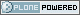# ICTP Portal

##### Sections
« October 2022 »
Su Mo Tu We Th Fr Sa
1
2 3 4 5 6 7 8
9 10 11 12 13 14 15
16 17 18 19 20 21 22
23 24 25 26 27 28 29
30 31

You are here: Home Insert a figure in LaTeX

# Insert a figure in LaTeX

Using graphics in LaTeX documents

If you have created a graphic with another program and want to include it in your document, try to produce an EPS file first. See the corresponding information in the chapters on Mathematica, Gnuplot, Xfig for instructions. If you are only able to create bitmap files (BMP, GIF, TIFF, etc.) you can use mogrify (see section File Formats) to convert them into PostScript.

• When you use LaTeX 2.09 (in other words, your document starts with the line documentstyle...), insert the following line at the beginning:
   \input ../images/epsf

At the point where you want to include the figure, write:
   \begin{figure}
\epsfbox{sin.eps}
\caption{The Sine function}
\end{figure}

Of course, you should replace the file name and the figure caption with the ones you need. You can also change the size of the figure using the commands
   \epsfxsize=5cm \epsfbox{sin.eps}

This will scale the figure to have a width of 5 cm.
• In LaTeX2e (where the first line of the document reads documentclass...) add the following line:
   \usepackage{epsfig}

At the point where you want to include the figure, put:
   \begin{figure}
\epsfig{file=sin.eps}
\caption{The Sine function}
\end{figure}

To scale the graphic and/or to rotate it, add the arguments width, height or angle as shown below:
   \epsfig{file=sin.eps,width=5cm,angle=90}

• In LaTeX2e, if you want the labels of an EPS figure to be TeXed, use the package psfrag. For more information, contact the help desk.This site conforms to the following standards: